# Equilibrium   Share

## What is Equilibrium

A state in which the rates of the forward and reverse reactions are equal and the concentrations of the reactants and products remain constant. Equilibrium is a dynamic process – the conversions of reactants to products and products to reactants are still going on, although there is no net change in the number of reactant and product molecules.

Take a reaction as follows:

Equilibrium Constant is given by the following equation:

Chemical equilibrium occurs when both the reactions(forward and backward) are occurring at equal rates.The chemical equilibrium and its laws have various real-life applications as mentioned below:

• Hemoglobin and oxygen exist in equilibrium in the blood by the reaction:
Hb(aq) + 4O2 Hb(O2)4(aq).
As a result, a person tends to feel light-headed at higher altitudes.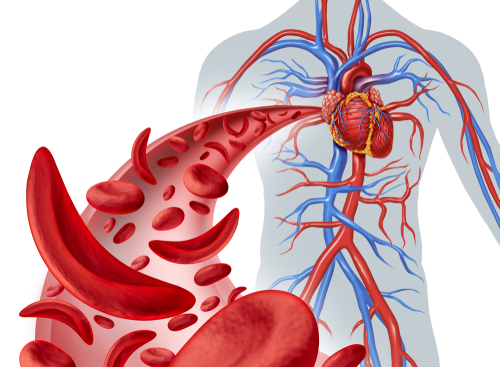Prepare Online for JEE Main/NEET

Crack JEE 2021 with JEE/NEET Online Preparation Program

• Nitrogen and other gases are dissolved in our blood, now if the diver comes up too fast from the deep ocean, suddenly there is an equilibrium shift takes place thus develops nitrogen bubbles in the blood which causes severe pain and death.## Some of the phase processes of equilibrium are as follows:

• Solid-liquid equilibrium:
For example, ice and water are equilibrium at only one temperature is called NORMAL MELTING POINT.

• Liquid-gas equilibrium:
For example, rate of evaporation = rate of condensation
H2O(l) ? H2O(vap)

• Solid-gas equilibrium:
This process is also called as sublimation
e.g. sublimation of camphor

Solid-liquid equilibrium:
Law of mass action: The equilibrium concentrations of reactants and products may vary, but the value Kc remains constant.

Characteristics of Kc

1. The reaction equilibrium can be obtained from both directions.

2. Kc is a function of temperature.

3. Kc is independent of the initial concentration of reactants and products.

4. If the Kc value is

• << 1, the equilibrium lies to the right and the reaction mixture contains mostly products.

• 0.10<Kc<10, the mixture contains proportionate amount reactants and products.

• >> 1, the equilibrium lies to the left and the reaction mixture contains mostly reactants.

Homogeneous Equilibria
When all the reactants and the products in the equilibrium are in the same phase, then this equilibrium is known as homogeneous equilibrium. For example:

In this reaction, all these reactants are in the gaseous phase, thus it is homogeneous equilibrium

Heterogeneous Equilibria
When the reactants are in different phases, then this system of equilibrium is known as the heterogeneous equilibria. For example,

In this reaction, all these reagents are in different phases, thus it is heterogeneous equilibrium.

• ## Predicts the extent of reaction, which gives the degree of the disappearance of reactants.

• Predicts the direction of the reaction.

• Calculating the equilibrium constant, which gives the relative amount of reactants and products.

Reaction Quotient (Q):
The reaction value obtained when we substitute reactant and product concentrations into the equilibrium expression.

1. If Q>K the reaction shifts towards the reactants side.

2. If Q<K the reaction shifts towards the products side.

3. If Q+K no shift occurs (equilibrium achieved).

## Factors affecting chemical equilibrium:

• Lechatlier ’s Principle: It states that changes in temperature, pressure, volume or concentration of a system will result in predictable and opposing changes in the system in order to achieve a new equilibrium state.It can be used to select the optimum conditions to form a substance.
• Changes in temperature:
Endothermic(H>0): R+ Heat Products.
Exothermic(h<0): R Products+ Heat
• Effect of catalyst:
The catalyst increases the rate of reaction for both forward and reverse reactions.The catalyst does not affect the equilibrium concentration of both reactants and products. So the value Kdoesn’t change.

## Ionic Equilibrium in Solution

Ionic equilibrium is the study of equilibrium between the ions. All those substances that are converted into ions in solution are classified into categories i.e, strong electrolytes and weak electrolytes. Strong electrolytes are those that are completely dissociated into ions and conduct greater electricity, while the weak electrolytes are those that are partially ionized in solutions and conduct smaller electricity. Acids, bases, and salts are either the weak electrolytes or strong electrolytes. In the case of weak electrolytes, the equilibrium established between the ions and non-ionized molecules.

## Ionization of Acids and Bases

As already mentioned, some acids and bases are strong in nature and some are weak in nature. When a strong acid dissociates in the aqueous medium then it forms its conjugate base, these conjugate bases are always weak in nature. Similarly, with strong bases, their conjugate acids in solutions are always weak. For weak acids and bases, their conjugates bases and acids, respectively are always strong in nature.

The acid-base dissociation equilibrium of an acid HA is given as below:

an acid-base conjugate acid conjugate base

## Ionic Product of Water

In pure water, one water molecule donates a proton and acts as an acid and another water molecule accepts a proton and acts as a base. The equilibrium reaction goes as follows:

The dissociation constant for this equilibrium reaction is given as follows:

can be eliminated since it is liquid, thus the ionic product of water or Kw is given as:

After calculations, the value of Kw is equal to = 1 x 10-14M2

Thus any aqueous solution is acidic, basic or neutral is determined by the conditions below:

• For acidic:
• For neutral:
• For basic:

## The pH Scale

The hydronium ion concentration of any solution is expressed in logarithmic scale, then it is known as pH scale. Thus, the pH of any solution is defined as the negative logarithm value of hydrogen ion concentration. Mathematically, it can be expressed as follows:

Now as we know, the pure water has hydrogen ion concentration = 10-7, therefore, pH of water = 7
Thus we can conclude as follows:

• Acidic solution has pH < 7
• Basic solution has pH > 7
• Neutral solution has pH = 7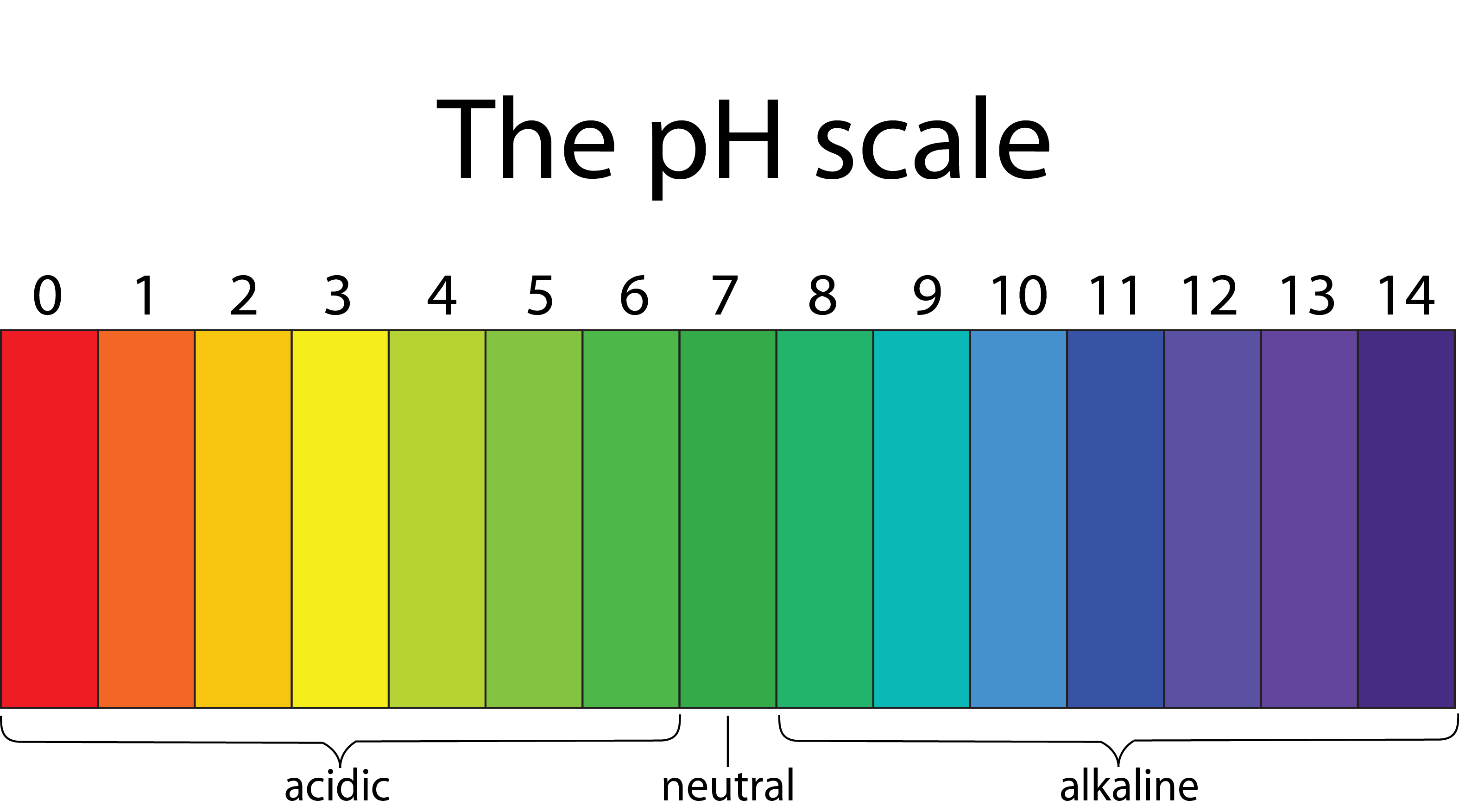From the above calculations, it has been found that:

## How to prepare for Equilibrium?

• This chapter is a part of Physical chemistry. This chapter is one of the most important chapters of the complete chemistry syllabus. Its concepts, laws, numerical and graphs all are important both for the basic foundation of chemistry and for scoring good marks in the examination.

• Before reading this chapter, first, you must have the basic knowledge of the mole concept.

• In this chapter, all the equations and formulae are very simple and easy to remember.

• Rest this chapter is very simple, just be regular and be consistent in your numerical practice.

## Prescribed Books for Equilibrium

First, you must finish the class XI NCERT textbook and solve each and every example and unsolved question given in it. Then for advanced level preparation like JEE and NEET, you must follow R.C. Mukherjee and O.P. Tandon. You must definitely solve the previous year papers. Meanwhile, in the preparation, you must continuously write the mock tests for the depth of knowledge. Our platform will help you to provide with the variety of questions for deeper knowledge with the help of videos, articles and mock tests.

## Chemistry Chapter- wise Notes for Engineering and Medical Exams

 Chapters No. Chapters Name Chapter 1 Some basic concepts in chemistry Chapter 2 States of matter Chapter 3 Atomic Structure Chapter 4 Solutions Chapter 5 Chemical Thermodynamics Chapter 7 Redox Reaction and Electrochemistry Chapter 8 Chemical kinetics Chapter 9 Surface Chemistry Chapter 10 General Principle and processes of Isolation of metals Chapter 11 Classification of Elements and Periodic table Chapter 12 Hydrogen Chapter 13 p- block Elements Chapter 14 s-block Elements (Alkali and Alkaline Earth Metals) Chapter 15 Chemical Bonding and Molecular Structure Chapter 16 d- and f- BLOCK ELEMENTS Chapter 17 Coordination Compounds Chapter  18 Environmental Chemistry Chapter 19 Purification and Characterisation of Organic Compounds Chapter 20 Some Basic Principles of Organic Chemistry Chapter 21 Hydrocarbons Chapter 22 Organic Compounds containing Halogens Chapter 23 Organic Compounds containing Oxygen Chapter 24 Organic Compounds Containing Nitrogen Chapter 25 Polymers Chapter 26 Biomolecules Chapter 27 Chemistry in Everyday Life Chapter 28 Principles Related to Practical Chemistry

### Topics from Equilibrium

• Meaning of equilibrium ( JEE Main ) (10 concepts)
• pressure, temperature, effect of catalyst ( JEE Main ) (2 concepts)
• factors affecting equilibrium concentration ( JEE Main ) (11 concepts)
• Equilibria involving physical processes: Solid -liquid, liquid - gas and solid - gas equilibria ( JEE Main ) (2 concepts)
• Henrys law ( JEE Main ) (1 concepts)
• Law of chemical equilibrium, ( JEE Main ) (6 concepts)
• equilibrium constants (Kp and Kc) and their significance ( JEE Main ) (9 concepts)
• hydrolysis of salts and pH of their solutions ( JEE Main ) (3 concepts)
• significance of Gibbs free energy in chemical equilibrium ( JEE Main ) (4 concepts)
• Le Chateliers principle ( JEE Main ) (1 concepts)
• Ionic equilibrium ( JEE Main ) (1 concepts)
• ionization of water, pH scale ( JEE Main ) (8 concepts)
• acid - base equilibria (including multistage ionization) and ionization constants ( JEE Main ) (12 concepts)
• various concepts of acids and bases (Arrhenius, Bronsted - Lowry and Lewis) and their ionization ( JEE Main ) (2 concepts)
• INTRODUCTION TO EQUILIBRIUM ( JEE Main ) (3 concepts)
• LAW OF CHEMICAL EQUILIBRIUM AND EQUILIBRIUM CONSTANT ( JEE Main ) (3 concepts)
• APPLICATIONS OF EQUILIBRIUM CONSTANTS ( JEE Main ) (3 concepts)
• FACTORS AFFECTING EQUILIBRIA ( JEE Main ) (2 concepts)
• IONIC EQUILIBRIUM ( JEE Main ) (1 concepts)
• ACIDS, BASES AND SALTS ( JEE Main ) (1 concepts)
• IONIZATION OF ACIDS AND BASES ( JEE Main ) (9 concepts)
• SOLUBILITY EQUILIBRIA OF SPARINGLY SOLUBLE SALTS ( JEE Main ) (6 concepts)
• BUFFER SOLUTIONS ( JEE Main ) (7 concepts)

### Important Books for Equilibrium

•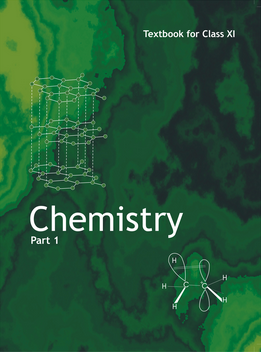•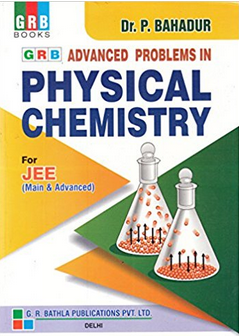•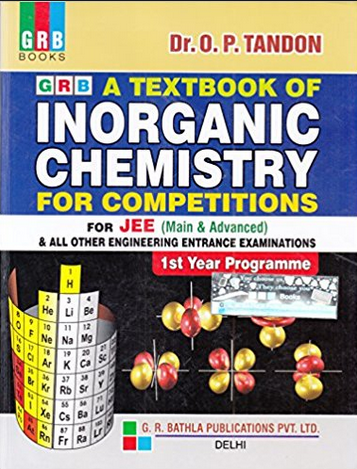Exams
Articles
Questions